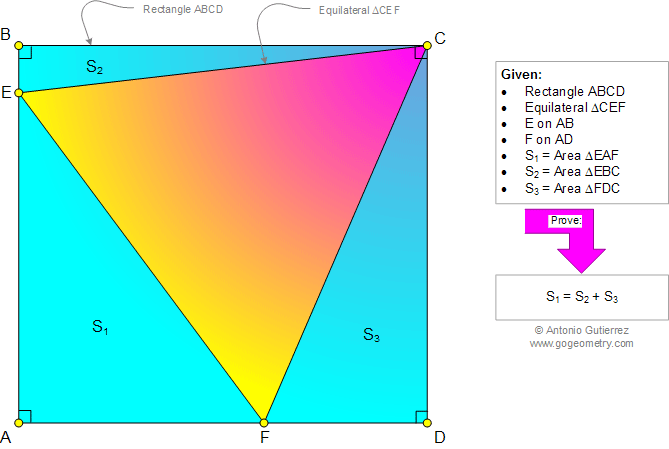# Geometry Problem 969: Equilateral Triangle, Rectangle, Common Vertex, Right Triangles Areas. Level: School, College, Mathematics Education

< PREVIOUS PROBLEM  |  NEXT PROBLEM >

 The figure shows a rectangle ABCD and equilateral triangle CEF (E on AB, F on AD). If S1, S2, and S3 are the areas of triangles EAF, EBC, and FDC, respectively, prove that S1 = S2 + S3.Home | Search | Geometry | Problems | All Problems | Open Problems | Visual Index | 10 Problems | Problems Art Gallery |  Art | 961-970 | Triangles | Equilateral Triangle | Rectangle | Areas | Right Triangle Area | by Antonio Gutierrez